# Samacheer Kalvi 6th Maths Solutions Term 3 Chapter 1 Fractions Ex 1.2## Tamilnadu Samacheer Kalvi 6th Maths Solutions Term 3 Chapter 1 Fractions Ex 1.2

Miscellaneous Practice Problems

Question 1.
Sankari purchased 2$$\frac{1}{2}$$ m cloth to stitch a long skirt and 1$$\frac{3}{4}$$ m cloth to stitch blouse. If the cost is ₹ 120 per metre then find the cost of cloth purchased by her
Solution:
Cloth to stitch a long skirt = 2$$\frac{1}{2}$$ m
Cloth to stitch a blouse = 1$$\frac{3}{4}$$ m
Total length of the cloth = $$2 \frac{1}{2}+1 \frac{3}{4} m=2+\frac{1}{2}+1+\frac{3}{4}$$ m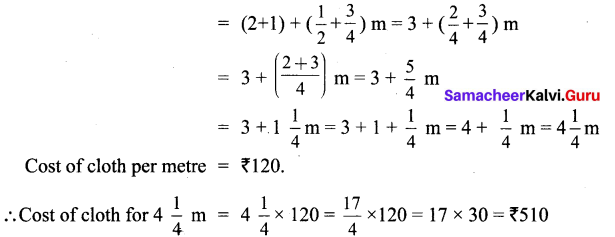Cost of cloth purchased by Sankari = ₹ 510

Question 2.
From his office, a person wants to reach his house on foot which is at a distance of 5$$\frac{3}{4}$$ km. If he had walked 2$$\frac{1}{2}$$ km, how much distance still he has to walk to reach his house?
Solution: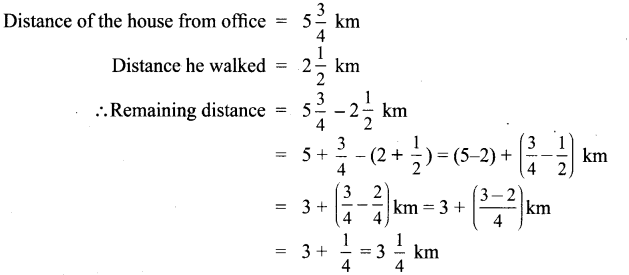Distance still he has to be walked = 3$$\frac{1}{4}$$ kmQuestion 3.
Which is smaller? The difference between $$\frac{1}{2}$$ and 3$$\frac{2}{3}$$ or the sum of 1$$\frac{1}{2}$$ and 2$$\frac{1}{4}$$
Solution: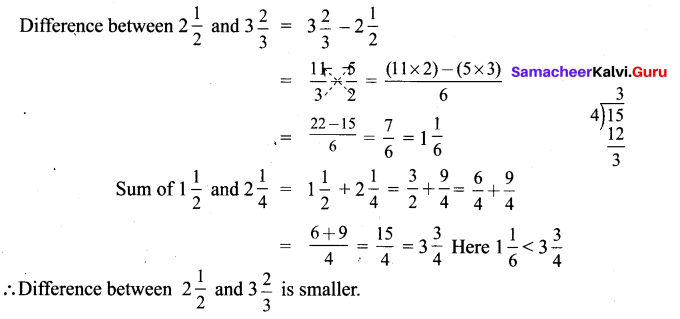Question 4.
Mangai bought 6$$\frac{3}{4}$$ kg of apples. If Kalai bought 1$$\frac{1}{2}$$ times as Mangai bought, then how many kilograms of apples did Kalai buy?
Solution:
Weight of apples Mangai bought = 6$$\frac{3}{4}$$ kg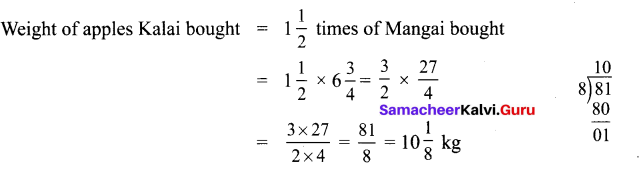Weight of apples Kalai bought = 10$$\frac{1}{8}$$ kg

Question 5.
The length of the staircase is 5 $$\frac{1}{2}$$ m. If one step is set at $$\frac{1}{4}$$ m, then how many steps will be there in the staircase?
Solution:
Length of the staircase = 5$$\frac{1}{2}$$ m
Distance between each step = $$\frac{1}{4}$$ m
∴ Number of steps in the staircase = $$5 \frac{1}{2} \div \frac{1}{4}=\frac{11}{2} \div \frac{1}{4}=\frac{11}{2} \times \frac{4}{1}$$ = 22
There will be 22 steps in the staircase

Challenge Problems

Question 6.
By using the following clues, find who am I?
(i) Each of my numerator and denominator is a single digit number.
(ii) The sum of my numerator and denominator is a multiple of 3.
(iii) The product of my numerator and denominator is a multiple of 4
Solution:
The numerator may be any one of!, 2, 3,4, 5, 6, 7, 8, 9 and the denominator may be any one of 1, 2,3,4, 5,6, 7,8,9. Sum of numerator and denominator is a multiple of 3.
∴ Possible proper fractions are $$\frac{1}{2}, \frac{1}{5}, \frac{1}{8}, \frac{2}{4}, \frac{2}{7}, \frac{3}{6}, \frac{3}{9}, \frac{4}{5}, \frac{4}{8}, \frac{5}{7}, \frac{6}{9}$$
Also given the product of numerator and denominator is a multiple of 4.
∴ Possible fractions are $$\frac{1}{8}, \frac{2}{4}, \frac{4}{5}, \frac{4}{8}$$

Question 7.
Add the difference between 1$$\frac{1}{3}$$ and 3$$\frac{1}{6}$$ and the difference between 4$$\frac{1}{6}$$ and 2$$\frac{1}{3}$$
Solution: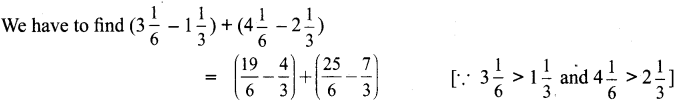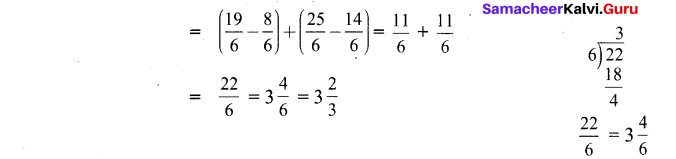Question 8.
What fraction is to be subtracted from 9$$\frac{3}{7}$$ to get 3$$\frac{1}{5}$$ ?
Solution: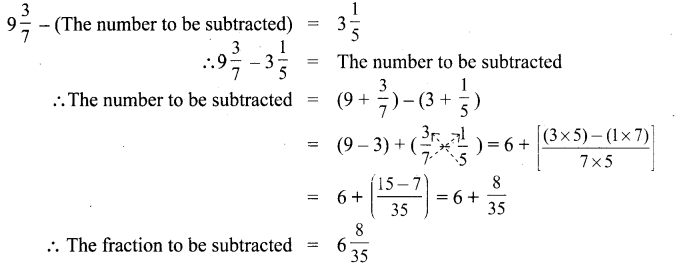Question 9.
The sum of two fractions is 5$$\frac{3}{9}$$. If one of the fractions is 2$$\frac{3}{4}$$, find the other fraction.
Solution: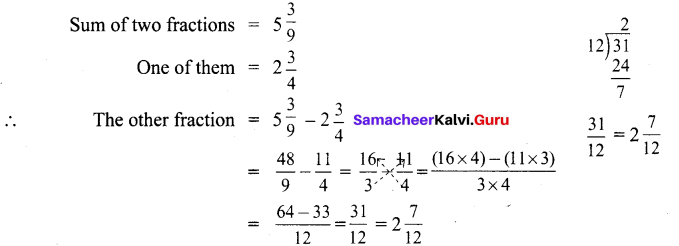The other number is 2$$\frac{7}{12}$$Question 10.
By what number should 3$$\frac{1}{16}$$ be multiplied to get 9$$\frac{3}{16}$$ ?
Solution: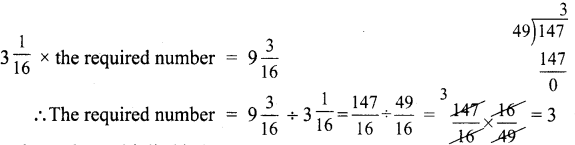The number to be multiplied is 3.

Question 11.
Complete the fifth row in the Leibnitz triangle which is based on subtraction.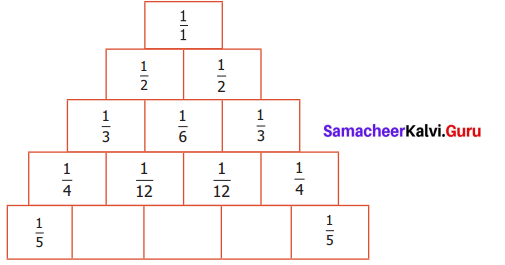Solution: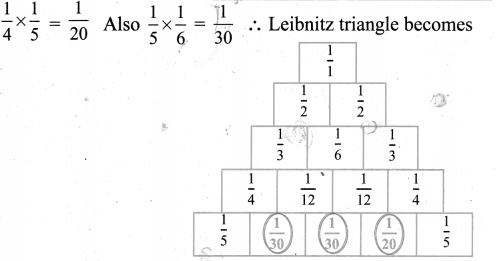Question 12.
A painted $$\frac{3}{8}$$ of the wall of which one third is painted in yellow colour. What fraction is the yellow colour of the entire wall.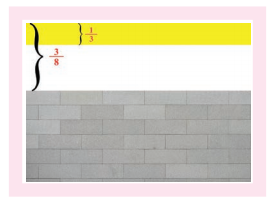Solution: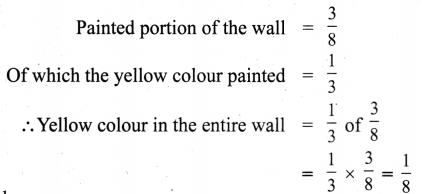$$\frac{1}{8}$$ of the wall is painted yellow.Question 13.
A rabbit has to cover 26$$\frac{1}{4}$$ m to fetch its food. If it covers 1$$\frac{3}{4}$$ m in one jump, thenhow many jumps will it take to fetch its food?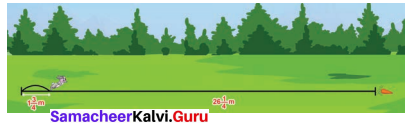Solution:
Total distance to be covered by the rabbit = 26$$\frac{1}{4}$$m
Distance covered in one jump = 1$$\frac{3}{4}$$ m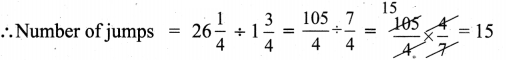∴ The rabbit jumps 15 times to fetch its food.

Question 14.
Look at the picture and answer the following questions :
(i) What is the distance from School to library via bus stop?
(ii) What is the distance between school and library via Hospital?
(iii) Which is the shortest distance between (i) and (ii)?
(iv) The distance between School and Hospital is ____ times the distance between school and Bus stop.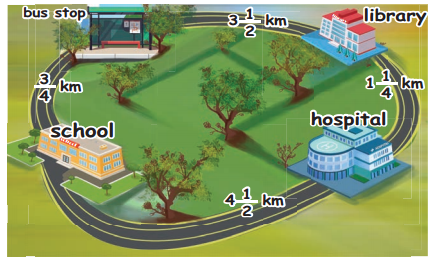Solution: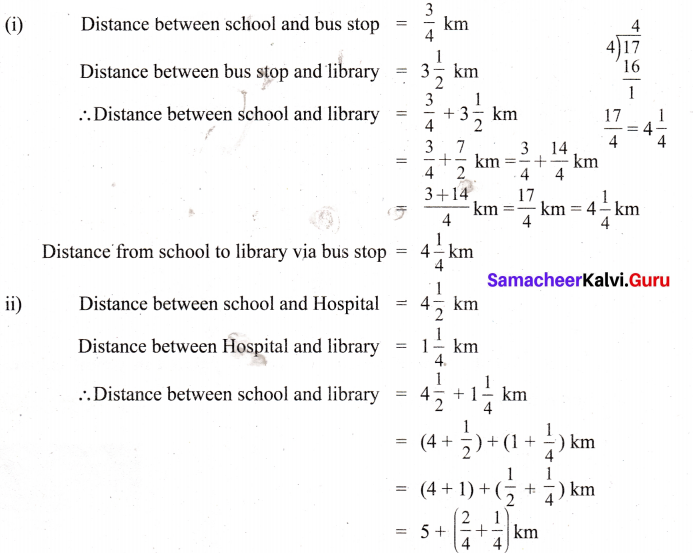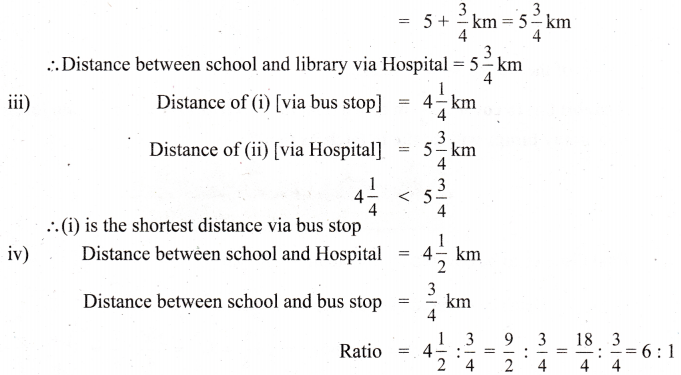The distance between school and Hospital is 6 times the distance between school and bus stop.